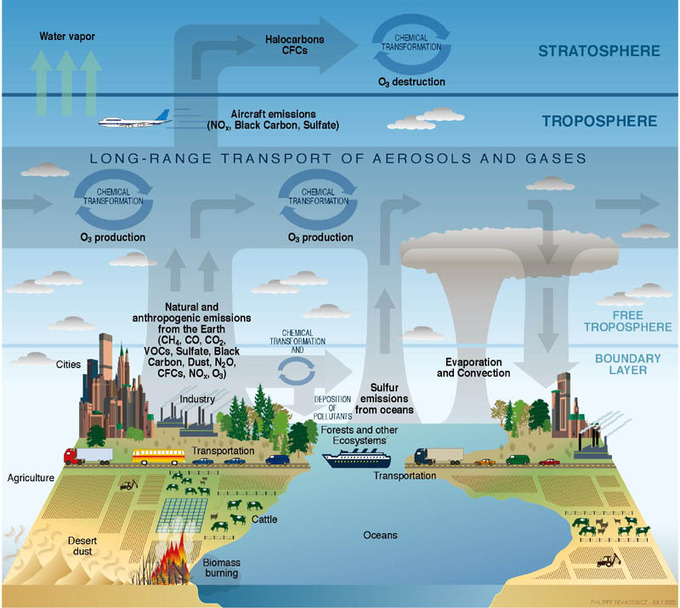## Density Calculations

#### Learning Objective

• Apply the reformulated Ideal Gas Equation in your calculations

#### Key Points

• Density calculations allow us to evaluate the behaviors of gases of unknown volume.
• We can determine the density of an ideal gas using knowledge of three properties of the evaluated ideal gas.
• This reformulation of the Ideal Gas Equation relates pressure, density, and temperature of an ideal gas independent of the volume or quantity of gas.

#### Term

• densitya measure of the amount of matter contained by a given volume

The Ideal Gas Equation in the form $PV=nRT$ is an excellent tool for understanding the relationship between the pressure, volume, amount, and temperature of an ideal gas in a defined environment that can be controlled for constant volume. However, in its most common form, the Ideal Gas Equation is not useful for examining the behavior of gases of undetermined volume, such as the gases in the clouds that surround the stars in our solar system or the atmospheric gases that support life on our planet. To derive a form of the ideal gas equation that has broader applications, we can use calculations that employ the physical property of density.Astronomical applications of the Ideal Gas LawThe Taurus Molecular Cloud consists of dust and various gases, including hydrogen and helium. The density form of the Ideal Gas Equation may be of theoretical use when studying such astronomical phenomena as star formation.Atmosphere compositionAtmospheric science offers one plausible real-life application of the density form of the ideal gas equation. Earth’s atmosphere is composed of gases that support life—as the image shows, oxygen involved in the water cycle, sulfur emissions from oceans, methane from agriculture, and more. The density form of the Ideal Gas Law enables us to study the behavior of these gases without enclosing them in a container of known volume.

# Derivation of the Volume-Independent Ideal Gas Law

We know the Ideal Gas Equation in the form $PV=nRT$. We also know that:

$n=\text{# moles of gas}=\frac{\text{mass of gas (m)}}{\text{molecular weight (M)}}=\frac{m}{M}$

If we substitute $\frac{m}{M}$ for n:

$PV= \frac{m}{M}RT$

Rearranging the above equation, we get:

$\frac{P}{RT}=\frac{m}{MV}$

Now, recall that density is equal to mass divided by volume:

$D=\frac{m}{V}$

The term $\frac{m}{V}$ appears on the right-hand side of the above rearranged Ideal Gas Law. We can substitute in density, D, and get the following:

$\frac{P}{RT}=\frac{D}{M}$

Rearranging in terms of D, we have:

$D=\frac{MP}{RT}$

This derivation of the Ideal Gas Equation allows us to characterize the relationship between the pressure, density, and temperature of the gas sample independent of the volume the gas occupies; it also allows us to determine the density of a gas sample given its pressure and temperature, or determine the molar mass of a gas sample given its density.